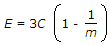# Civil Engineering - Strength of Materials

### Exercise :: Strength of Materials - Section 3

6.

The shape of the bending moment diagram over the length of a beam, carrying a uniformly increasing load, is always

 A. linear B. parabolic C. cubical D. circular.

Explanation:

No answer description available for this question. Let us discuss.

7.

When a rectangular beam is loaded longitudinally, shear develops on

 A. bottom fibre B. top fibre C. middle fibre D. every-horizontal plane.

Explanation:

No answer description available for this question. Let us discuss.

8.

The tension coefficient of any member is

 A. force divided by the length B. tension divided by the length C. tension per unit area D. tension in the member.

Explanation:

No answer description available for this question. Let us discuss.

9.

A steel rod of 2 cm diameter and 5 metres long is subjected to an axial pull of 3000 kg. If E = 2.1 x 106, the elongation of the rod will be

 A. 2.275 mm B. 0.2275 mm C. 0.02275 mm D. 2.02275 mm.

Explanation:

No answer description available for this question. Let us discuss.

10.

For a given material, if E, C, K and m are Young's modulus, shearing modulus, bulk modulus and poisson ratio, the following relation does not hold good

 A.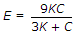B.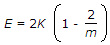C.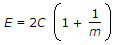D.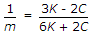E.